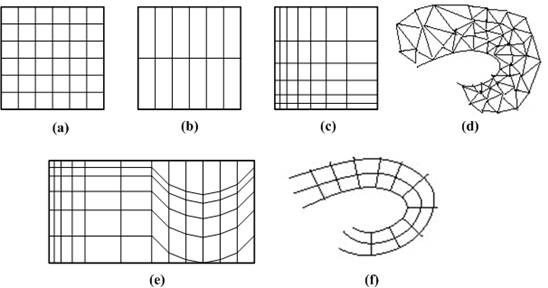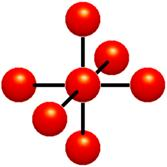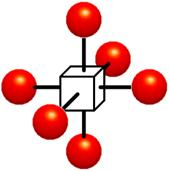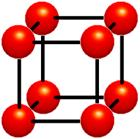# 1  Volume Rendering

## 1.1Volume Rendering

Rapid advances in hardware have been transforming revolutionary approaches in computer graphics into reality. One typical example is the raster graphics that took place in the seventies, when hardware innovations enabled the transition from vector graphics to raster graphics. Another example, which has a similar potential, is currently shaping up in the field of volume graphics. This trend is rooted in the extensive research and development effort in scientific visualization in general and in volume visualization in particular.

Visualization is the usage of computer-supported, interactive, visual representations of data to amplify cognition. Scientific visualization is the visualization of physically based data. Volume visualization is a method of extracting meaningful information from volumetric datasets through the use of interactive graphics and imaging, and is concerned with the representation, manipulation, and rendering of volumetric datasets. Its objective is to provide mechanisms for peering inside volumetric datasets and to enhance the visual understanding.

Traditional 3D graphics is based on surface representation. Most common form is polygon-based surfaces for which affordable special-purpose rendering hardware have been developed in the recent years. Volume graphics has the potential to greatly advance the field of 3D graphics by offering a comprehensive alternative to conventional surface representation methods.

Our display screens are composed of a two-dimensional array of pixels each representing a unit area. A volume is a three-dimensional array of cubic elements, each representing a unit of space. Individual elements of a three-dimensional space are called volume elements or voxels. A number associated with each point in a volume is called the value at that point. The collection of all these values is called a scalar field on the volume. The set of all points in the volume with a given scalar value is called a level surface. Volume rendering is the process of displaying scalar fields. It is a method for visualizing a three-dimensional data set. The interior information about a data set is projected to a display screen using the volume rendering methods. Along the ray path from each screen pixel, interior data values are examined and encoded for display. How the data are encoded for display depends on the application. Seismic data, for example, is often examined to find the maximum and minimum values along each ray. The values can then be color coded to give information about the width of the interval and the minimum value. In medical applications, the data values are opacity factors in the range from 0 to 1 for the tissue and bone layers. Bone layers are completely opaque, while tissue is somewhat transparent. Voxels represent various physical characteristics, such as density, temperature, velocity, and pressure. Other measurements, such as area, and volume, can be extracted from the volume datasets. Applications of volume visualization are medical imaging (e.g., computed tomography, magnetic resonance imaging, ultrasonography), biology (e.g., confocal microscopy), geophysics (e.g., seismic measurements from oil and gas exploration), industry (e.g., finite element models), molecular systems (e.g., electron density maps), meteorology (e.g., stormy (prediction), computational fluid dynamics (e.g., water flow), computational chemistry (e.g., new materials), digital signal and image processing (e.g., CSG ). Numerical simulations and sampling devices such as magnetic resonance imaging (MRI), computed tomography (CT), positron emission tomography (PET), ultrasonic imaging, confocal microscopy, supercomputer simulations, geometric models, laser scanners, depth images estimated by stereo disparity, satellite imaging, and sonar are sources of large 3D datasets.

3D scientific data can be generated in a variety of disciplines by using sampling methods. Volumetric data obtained from biomedical scanners typically come in the form of 2D slices of a regular, Cartesian grid, sometimes varying in one or more major directions. The typical output of supercomputer and Finite Element Method (FEM) simulations is irregular grids. The raw output of an ultrasound scan is a sequence of arbitrarily oriented, fan-shaped slices, which constitute partially structured point samples. A sequence of 2D slices obtained from these scanners is reconstructed into a 3D volume model. Imaging machines have a resolution of millimeters scale so that many details important for scientific purposes can be recorded.

It is often necessary to view the dataset from continuously changing positions to better understand the data being visualized. The real-time interaction is the most essential requirement and preferred even if it is rendered in a somewhat less realistic way. A real-time rendering system is important for the following reasons:

·        to visualize rapidly changing datasets,

·        for real-time exploration of 3D datasets, (e.g. virtual reality)

·        for interactive manipulation of visualization parameters, (e.g. classification)

·        for interactive volume graphics.

Rendering and processing does not depend on the object’s complexity or type, it depends only on volume resolution. The dataset resolutions are generally anywhere from 1283 to 10243 and may be non-symmetric (i.e. 1024 x 1024 x 512).

## 1.2Volumetric Data

Volumetric data is typically a set of samples S(x, y, z, v), representing the value v of some property of the data, at a 3D location (x, y, z). If the value is simply a 0 or a 1, with a value of 0 indicating background and a value of 1 indicating the object, then the data is referred to as binary data. The data may instead be multi-valued, with the value representing some measurable property of the data, including, for example, color, density, heat or pressure. The value v may even be a vector, representing, for example, velocity at each location. In general, the samples may be taken at purely random locations in space, but in most cases the set S is isotropic containing samples taken at regularly spaced intervals along three orthogonal axes. When the spacing between samples along each axis is a constant, then S is called isotropic, but there may be three different spacing constants for the three axes. In that case the set S is anisotropic. Since the set of samples is defined on a regular grid, a 3D array (called also volume buffer, cubic frame buffer, 3D raster) is typically used to store the values, with the element location indicating position of the sample on the grid. For this reason, the set S will be referred to as the array of values S(x, y, z), which is defined only at grid locations. Alternatively, either rectilinear, curvilinear (structured), or unstructured grids, are employed (Figure 1‑1).Figure 1‑1: Grid types in volumetric data. a. Cartesian grid, b. Regular grid, c. Rectilinear grid, d. Curvilinear grid, e. Block structured grid, and   f. Unstructured grid

In a rectilinear grid the cells are axis-aligned, but grid spacing along the axes are arbitrary. When such a grid has been non-linearly transformed while preserving the grid topology, the grid becomes curvilinear. Usually, the rectilinear grid defining the logical organization is called computational space, and the curvilinear grid is called physical space. Otherwise the grid is called unstructured or irregular. An unstructured or irregular volume data is a collection of cells whose connectivity has to be specified explicitly. These cells can be of an arbitrary shape such as tetrahedra, hexahedra, or prisms.

The array S only defines the value of some measured property of the data at discrete locations in space. A function f(x, y, z) may be defined over the volume in order to describe the value at any continuous location. The function f(x, y, z) = S(x, y, z) if (x, y, z) is a grid location, otherwise f(x, y, z) approximates the sample value at a location (x, y, z) by applying some interpolation function to S. There are many possible interpolation functions. The simplest interpolation function is known as zero-order interpolation, which is actually just a nearest-neighbor function. The value at any location in the volume is simply the value of the closest sample to that location. With this interpolation method there is a region of a constant value around each sample in S. Since the samples in S are regularly spaced, each region is of a uniform size and shape. The region of the constant value that surrounds each sample is known as a voxel with each voxel being a rectangular cuboid having six faces, twelve edges, and eight corners.

Higher-order interpolation functions can also be used to define f(x, y, z) between sample points. One common interpolation function is a piecewise function known as first-order interpolation, or trilinear interpolation. With this interpolation function, the value is assumed to vary linearly along directions parallel to one of the major axes.

## 1.3Voxels and cells

Volumes of data are usually treated as either an array of voxels or an array of cells. These two approaches stem from the need to resample the volume between grid points during the rendering process. Resampling, requiring interpolation, occurs in almost every volume visualization algorithm. Since the underlying function is not usually known, and it is not known whether the function was sampled above the Nyquist frequency, it is impossible to check the reliability of the interpolation used to find data values between discrete grid points. It must be assumed that common interpolation techniques are valid for an image to be considered valid.Figure 1‑2: Voxels. Each grid point has a sample value. Data values do not vary within voxels.

The voxel approach dictates that the area around a grid point has the same value as the grid point (Figure 1‑2). A voxel is, therefore, an area of non-varying value surrounding a central grid point. The voxel approach has the advantage that no assumptions are made about the behavior of data between grid points; only known data values are used for generating an image.Figure 1‑3 : Cells. Data values do vary within cells. It is assumed that values between grid points can be estimated. Interpolation is used

The cell approach views a volume as a collection of hexahedra whose corners are grid points and whose value varies between the grid points (Figure 1‑3). This technique attempts to estimate values inside the cell by interpolating between the values at the corners of the cell. Trilinear and tricubic are the most commonly used interpolation functions. Images generated using the cell approach, appear smoother than those images created with the voxel approach. However, the validity of the cell-based images cannot be verified.

## 1.4Problems Associated with Volume Rendering

As mentioned before, volume visualization is the technique of displaying two-dimensional projections of three-dimensional (volume) data. Data may be acquired from various scanners, such as an MRI, CT, SPECT, or US scanner. Visualizing a given three dimensional dataset can be done using two different approaches based on the portion of the volume raster set which they render; surface rendering, and volume rendering. Surface rendering algorithms first fit geometric primitives to values in the data, and then render these primitives. Since surface rendering algorithms require an intermediate representation, they are less attractive. Volume rendering algorithms render voxels in the volume raster directly, without converting to geometric primitives or first converting to a different domain and then rendering from that domain. They usually include an illumination model, which supports semi-transparent voxels.

Volume rendering can produce informative images that can be useful in data analysis, but a major drawback of the techniques is the time required to generate a high-quality image. An interactive volume visualization scheme requires a performance in the order of Terra (1012) operations per second. General-purpose processors alone cannot provide such a performance, and so, additional solutions must be explored. Several volume rendering optimizations have been developed which decrease rendering times, and therefore increase interactivity and productivity. For the optimization of volume visualization there are five main approaches: data reduction by means of model extraction or data simplification, software-based algorithm optimization and acceleration, implementation on general purpose parallel architectures, use of contemporary off-the-shelf graphics hardware, and realization of special-purpose volume rendering engines.

Software based optimizations are divided into two broad groups according to the rendering methods they use, that is, optimization methods for object-space rendering, and image-space rendering. The image-space optimization methods are based on the observation that most of the existing methods for speeding up the process of ray casting rely on one or more of the following principles: pixel-space coherency, object-space coherency, inter-ray coherency, frame coherency, space-leaping, vertical coherency, temporal coherency, and stereoscopic coherency.

The most effective branch of volume rendering acceleration techniques involve the utilization of the fifth principle: speeding up ray casting by providing efficient means to traverse the empty space, that is space leaping . The passage of a ray through the volume is two phased. In the first phase, the ray advances through the empty space searching for an object. In the second phase, the ray integrates colors and opacities as it penetrates the object. Since the passage of empty space does not contribute to the final image, skipping the empty space provides a significant speed up without affecting the image quality.

The optimization techniques will be examined in more detail in the next chapter.